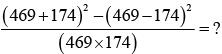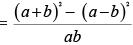Courses

## 10 Questions MCQ Test Maths Olympiad Class 6 | Olympiad Test : Simplification

Description
This mock test of Olympiad Test : Simplification for Class 6 helps you for every Class 6 entrance exam. This contains 10 Multiple Choice Questions for Class 6 Olympiad Test : Simplification (mcq) to study with solutions a complete question bank. The solved questions answers in this Olympiad Test : Simplification quiz give you a good mix of easy questions and tough questions. Class 6 students definitely take this Olympiad Test : Simplification exercise for a better result in the exam. You can find other Olympiad Test : Simplification extra questions, long questions & short questions for Class 6 on EduRev as well by searching above.
QUESTION: 1

### David gets on an elevator at the 11th floor of a building and rides up at the rate of 57 floors per minute. At the same time, Albert gets on an elevator at the 51st floor of the same building and rides down at the rate of 63 floors per minute. If they continue travelling at these rates, then at which floor will their paths cross?

Solution:

Suppose their paths cross after x minutes.
Then, 11 + 57x = 51 – 63x
⇒ 120x = 40
⇒ x =1/3
Number of floors covered by David in (1/3) min. =1/3 × 57= 19.
So, their paths cross at (11 +19) i.e., 30th floor.

QUESTION: 2

###Solution:

Let a = 469
b = 469 there
given expression=  4ab/ab
=  4

QUESTION: 3

### A man has some hens and cows. If the number of heads be 48 and the number of feet equals 140, then the number of hens will be:

Solution:

Let the number of hens be x and the number of cows be y.
Then, x + y = 48.... (i) and 2x + 4y = 140
⇒ x + 2y = 70 .... (ii)
Solving (i) and (ii) we get: x = 26, y = 22.
∴ The required answer = 26.

QUESTION: 4

Free notebooks were distributed equally among children of a class. The number of notebooks each child got was one-eighth of the number of children. Had the number of children been half, each child would have got 16 notebooks. Total how many notebooks were distributed?

Solution:

Let total number of children be x.
Then, x × (1/8) x = x/2 × 16  ⇔ x = 64
∴ Number of notebooks = x2/8
=1/8 × 64 × 64 = 512

QUESTION: 5

In a regular week, there are 5 working days and for each day, the working hours are 8. A man gets Rs 2.40 per hour for regular work and Rs 3.20 per hours for overtime. If he earns Rs 432 in 4 weeks, then how many hours does he work for?

Solution:

Suppose the man works overtime for x hours.
Now, working hours in 4 weeks = (5 × 8 × 4) = 160
∴ 160 × 2.40 + x × 3.20 = 432
⇒ 3.20x = 432 – 384 = 48
⇒ x = 15
Hence, total hours of work = (160 + 15) = 175

QUESTION: 6

To fill a tank, 25 buckets of water are required. How many buckets of water will be required to fill the same tank if the capacity of the bucket is reduced to two-fifth of its present?

Solution:

Let the capacity of 1 bucket = x
Then, the capacity of tank = 25x
New capacity of bucket = 2x/5
∴ Required number of buckets
= 25x/ (2x/5) =125/2 = 62.5

QUESTION: 7

Eight people are planning to share equally the cost of a rental car. If one person withdraws from the arrangement and the others share equally the entire cost of the car, then the share of each of the remaining persons is increased by:

Solution:

Original share of 1 person =1/8
New share of 1 person =1/7
Increase = 1/7 – 1/8 = 1/56
∴ Required fraction = (1/56) / (1/8) = 1/7

QUESTION: 8

A fires 5 shots to B’s 3 but A kills only once in 3 shots while B kills once in 2 shots. When B has missed 27 times, A has killed:

Solution:

Let the total number of shots be x. Then,
Shots fired by A    = 5x/8
Shots fired by B    = 3x/8
Killing shots by A = 1/3 of 5x/8 = 5x/24
Shots missed by B = 1/2 of 3x/8 = 3x/16
∴ 3x/16= 27 or x = 144
Birds killed by A = 5x/24 = (5x 144)/24 = 30

QUESTION: 9

One-third of Rahul’s savings in National Savings Certificate is equal to one-half of his savings in Public Provident Fund. If he has Rs 1, 50,000 as total savings, how much has he saved in Public Provident Fund?

Solution:

Let savings in N.S.C and P.P.F. be Rs x and Rs.
(150000 – x) respectively. Then,
1/3x =1/2(150000 – x)
⇒ x/3 + x/2 = 75000
⇒ 5x/6 = 75000
⇒ x = (75000 × 6)/5 = Rs = 90000
∴ Savings in Public Provident Fund
= Rs (1 50000 – 90000) = Rs 60000

QUESTION: 10

A sum of Rs 1360 has been divided among A, B and C such that A gets 2/3 of what B gets and B gets 1/4 of what C gets. B’s share is:

Solution:

Let C’s share = Rs x
Then, B’s share = Rs x/4,
A’s share = Rs 2/3 × x/4    = Rs x/6
⇒ 17x/12 = 1360
⇒ x = (1360 × 12)/17= Rs 960
Hence, B’s share = Rs 960/4 = Rs 240